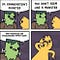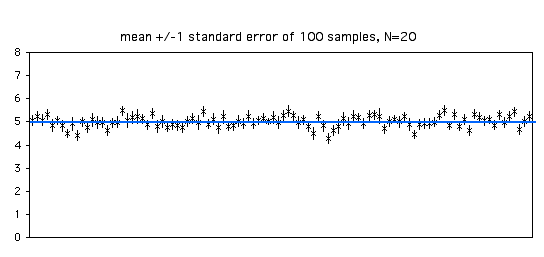# Test statistics and effect sizes

## The two statistical concepts are distinct.

Researchers may confuse a test statistic for a standardised effect size.

Test statistics and standardised effect sizes are distinct. The two figures may have similar formulae, but represent different things. This article shows the example of comparing mean averages in independent samples.(Image: Patrick English/Twitter)

# Spot the difference

Researchers are often interested in how two independent samples are different from another. For example, they may wish to compare the efficacy of a drug against a competitor or placebo.

What is the difference between test statistics and effect sizes? Here, I compare the t-stat (a test statistic) and Cohen’s d (a standardised effect size).

You do not need to understand mathematical formulae for this part. You only need to be able to spot differences. This is the t-stat:

This is the formula for Cohen’s d:

In both cases, I have used the pooled standard deviation from both samples.

There are two critical differences.

## Missing mu

The Greek letters on the top half of the fraction are not present in the second stat. In a t-test, this represents the (hypothetical) difference in the population means. This is usually zero, representing a null hypothesis of no difference.

We care about the arithmetic difference in sample means minus our hypothetical difference. How different are the samples to our hypothesis?

When we calculate the effect size, we care about the difference. As the name suggests, it is the effect that interests us.

## Denominator

The bottom term in the t-stat formula is much smaller for large samples.

Whilst the formulae have similar shapes, the two statistics are different.

# Standard deviations and standard errors

There is sometimes confusion among researchers between standard deviations and standard errors.(Image: BBC America/Giphy)

The effect size represents how different are the two sample means from each other. How big is the effect? The calculation uses the sample standard deviation. That measures how much each unit within the sample differ from its mean average.

The test statistic is different. If we drew many samples, how often would we observe that scale of difference or something even bigger?

Imagine there were others (like in a multiverse) doing the exact same test with the same study design.

From each pair of samples, we could calculate the difference in sample means. How much would the difference in sample means vary? This is about the variance between samples.

We could draw any number of these samples. The theoretical distribution that these samples come from is the sampling distribution.

The standard error is the standard deviation of this sampling distribution.Every sample drawn has a mean. How much do these sample means vary? (Image: J. H. McDonald)

The sample standard deviation is different to the standard error.

The former measures variation within samples whilst the latter measures variation between samples. As the sample sizes increase, a random sample will have smaller standard errors. This leads to more precise estimates.

That is why the t-stat and Cohen’s d differ. A test statistic and standardised effect sizes are trying to answer different questions.

This blog looks at the use of statistics in Britain and beyond. It is written by RSS Statistical Ambassador and Chartered Statistician @anthonybmasters.

## More from Anthony B. Masters

This blog looks at the use of statistics in Britain and beyond. It is written by RSS Statistical Ambassador and Chartered Statistician @anthonybmasters.